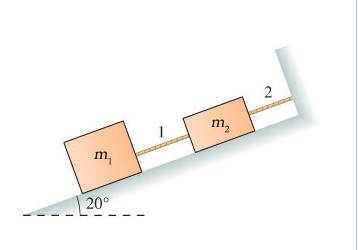# Problem: Two blocks are at rest on a frictionless incline, as shown in (Figure 1).What is the tension in the string number 1 if m1 = 9.0 kg and m2 = 3.0 kg ?What is the tension in the string number 2 if m1 = 9.0 kg and m2 = 3.0 kg ?

###### FREE Expert Solution

Newton's second law:

$\overline{){\mathbf{\Sigma }}{\mathbf{F}}{\mathbf{=}}{\mathbf{m}}{\mathbf{a}}}$

(a)

Take: y-axis points to the up and x-axis points to the left.

We'll apply Newton's second law to the forces parallel to the incline:

ΣF = T1 - m1gcosθ = 0

84% (340 ratings)###### Problem Details

Two blocks are at rest on a frictionless incline, as shown in (Figure 1).What is the tension in the string number 1 if m1 = 9.0 kg and m2 = 3.0 kg ?

What is the tension in the string number 2 if m1 = 9.0 kg and m2 = 3.0 kg ?